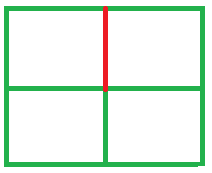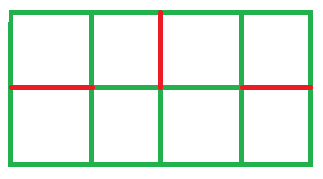# Minimum Cuts can be made in the Chessboard such that it is not divided into 2 parts

Given M x N Chessboard. The task is to determine the Maximum numbers of cuts that we can make in the Chessboard such that the Chessboard is not divided into 2 parts.

Examples:

```Input: M = 2, N = 4
Output: Maximum cuts = 3

Input: M = 3, N = 3
Output: Maximum cuts = 4
```

## Recommended: Please try your approach on {IDE} first, before moving on to the solution.

Representation:

1. For M = 2, N = 2 We can only make 1 cut (mark in red). if we make 1 more cut then the chessboard will divide into 2 pieces.
2.3. For M = 2, N = 4 We can makes 3 cuts (marks in red). if we make 1 more cut then the chessboard will divide into 2 pieces.
4.So, it can be observed that no. of cuts = (m-1) * (n-1).

Below is the implementation of the above approach:

## C++

 `// C++ implementation of above approach ` `#include ` `using` `namespace` `std; ` ` `  `// function that calculates the ` `// maximum no. of cuts ` `int` `numberOfCuts(``int` `M, ``int` `N) ` `{ ` `    ``int` `result = 0; ` ` `  `    ``result = (M - 1) * (N - 1); ` ` `  `    ``return` `result; ` `} ` ` `  `// Driver Code ` `int` `main() ` `{ ` `    ``int` `M = 4, N = 4; ` ` `  `    ``// Calling function. ` `    ``int` `Cuts = numberOfCuts(M, N); ` ` `  `    ``cout << ``"Maximum cuts = "` `<< Cuts; ` ` `  `    ``return` `0; ` `} `

## Java

 `// Java implementation of above approach ` ` `  `class` `GFG { ` `     `  `// function that calculates the ` `// maximum no. of cuts ` `static` `int` `numberOfCuts(``int` `M, ``int` `N) ` `{ ` `    ``int` `result = ``0``; ` ` `  `    ``result = (M - ``1``) * (N - ``1``); ` ` `  `    ``return` `result; ` `} ` ` `  `// Driver Code ` `public` `static` `void` `main(String args[]) ` `{ ` `    ``int` `M = ``4``, N = ``4``; ` ` `  `    ``// Calling function. ` `    ``int` `Cuts = numberOfCuts(M, N); ` ` `  `    ``System.out.println(``"Maximum cuts = "` `+ Cuts); ` ` `  `} ` `} `

## Python3

 `# Python3 implementation of  ` `# above approach ` ` `  `# function that calculates the ` `# maximum no. of cuts ` `def` `numberOfCuts(M, N): ` `    ``result ``=` `0` `     `  `    ``result ``=` `(M ``-` `1``) ``*` `(N ``-` `1``) ` `     `  `    ``return` `result ` ` `  `# Driver code ` `if` `__name__``=``=``'__main__'``: ` `     `  `    ``M, N ``=` `4``, ``4` `     `  `    ``# Calling function. ` `    ``Cuts ``=` `numberOfCuts(M, N) ` `     `  `    ``print``(``"Maximum cuts = "``, Cuts) ` ` `  `# This code is contributed by ` `# Kriti_mangal `

## C#

 `//C#  implementation of above approach  ` `using` `System; ` ` `  `public` `class` `GFG{ ` `// function that calculates the  ` `// maximum no. of cuts  ` `static` `int` `numberOfCuts(``int` `M, ``int` `N)  ` `{  ` `    ``int` `result = 0;  ` ` `  `    ``result = (M - 1) * (N - 1);  ` ` `  `    ``return` `result;  ` `}  ` ` `  `// Driver Code  ` `     `  `    ``static` `public` `void` `Main (){ ` `     `  `    ``int` `M = 4, N = 4;  ` `    ``// Calling function.  ` `    ``int` `Cuts = numberOfCuts(M, N);  ` ` `  `    ``Console.WriteLine(``"Maximum cuts = "` `+ Cuts);  ` `    ``} ` `//This code is contributed by akt_mit     ` `} `

## PHP

 ` `

Output:

```Maximum cuts = 9
```

Attention reader! Don’t stop learning now. Get hold of all the important DSA concepts with the DSA Self Paced Course at a student-friendly price and become industry ready.

My Personal Notes arrow_drop_upCheck out this Author's contributed articles.

If you like GeeksforGeeks and would like to contribute, you can also write an article using contribute.geeksforgeeks.org or mail your article to contribute@geeksforgeeks.org. See your article appearing on the GeeksforGeeks main page and help other Geeks.

Please Improve this article if you find anything incorrect by clicking on the "Improve Article" button below.

Improved By : Kirti_Mangal, jit_t, AnkitRai01